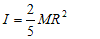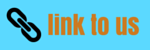# Rotation

## (5)Calculation of moment of inertia

• We already know that the moment of inertia of a system about axis of rotation is given as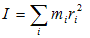where mi is the mass of the ith particle and ri is its perpendicular distance from the axis of rotation
• For a system consisting of collection of discrete particles ,above equation can be used directly for calculating the moment of inertia
• For continuous bodies ,moment of inertia about a given line can be obtained using integration technique
• For this imagine dividing entire volume of the rigid body into small volume elements dV so that all the points in a particular volume element are approximately at same distance from the axis of rotation and le r be this distance
• if dm is the mass of this volume element dV,then moment of inertia may be given by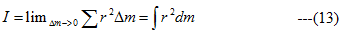• Since density ρ of the element is defined as mass per unit volume so ρ=dm/dV hence equation (13) may be written as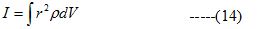• For homogeneous body density ρ is uniform hence ρ can be taken out of the integral sign i.e.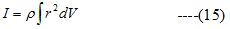above integration can be carried out easily for bodies having regular shapes as can be seen from examples given below
i) Moment of inertia of uniform rod about a perpendicular bisector
• Consider a homogeneous and uniform rod of mass M and length L as shown below in the figure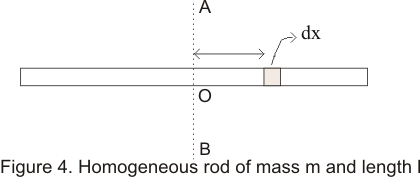• we have to calculate the moment of inertia of the rod about the bisector AB
• Consider middles point O to be the origin of the rod .Also consider an element of the rod between the distance x and x+dx from the origin. Since the rod is uniform so its density
ρ=M/L
Hence mass of the element dm=(M/L)dx
• Perpendicular distance of this element from line AB is x,so that moment of inertia of this element about AB is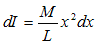• For x=-L/2,the element is at the left end of the rod ,As x changes from -L/2 to +L/2 ,the element covers the whole rod
• Thus the moment of inertia of the entire rod about AB is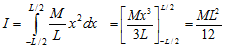ii) Moment of inertia of the uniform circular plate about its axis
• Consider a uniform circular plate of mass M and radius R as shown below in the figure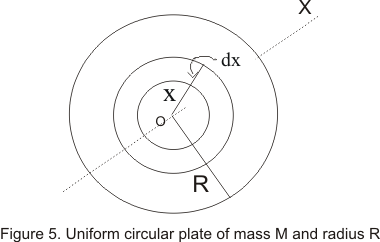• Let O be the center of the plate and OX is the axis perpendicular to the plane of the paper
• To find the moment of inertia of the plate about the axis OX draw two concentric circles of radii x and x+dx having these centers at O,so that they form a ring
• Area of this ring is equal to its circumference multiplied by its width i.e.
Area of the ring =2πxdx
• Mass of the ring would be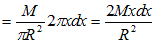• Moment of inertia of this ring about axis OX would be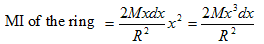• Since whole disc can be supposed to be made up of such like concentric rings of radii ranging from O to R ,we can find moment of inertia I of the disc by integrating moment of inertia of the ring for the limits x=0 and x=R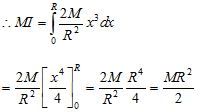iii) Moment of inertia of a uniform sphere of radius R about the axis through its center
• Consider a sphere of mass M and radius R .Let us divide this sphere into thin discs as shown in the figure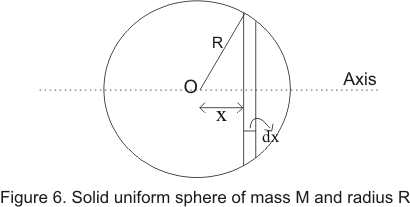• If r is the distance of the disc then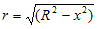• Volume of the disc would be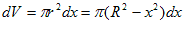and its mass would be
dm=ρdV
• Moment of inertia of this disc would be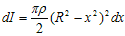Moment of inertia of the whole sphere would be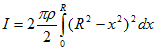Factor 2 appears because of symmetry considerations as the right hemisphere has same MI as that of left one
• Integration can be carried out easily by expanding (R2-x2)2 .On integrating above equation we find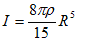Now mass of the sphere is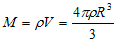Hence